# LevelSet¶

class LevelSet(*args)

Level set.

Available constructors:

LevelSet(dim=1)

LevelSet(function=ot.SymbolicFunction([‘x’], [‘1.0’]), operator=ot.LessOrEqual(), level=0.0)

Parameters:
dimint,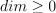Dimension of the LevelSet.

functionFunction

A function such that: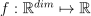defining the LevelSet.

operatorComparisonOperator

Comparison operator against the level.

levelfloat

Leveldefining the LevelSet.

Notes

A LevelSet is a Domain defined as follows: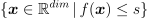Examples

>>> import openturns as ot
>>> function = ot.SymbolicFunction(['x1', 'x2'], ['x1^4 + x2^4'])
>>> s = 1.0
>>> op = ot.LessOrEqual()
>>> levelSet = ot.LevelSet(function, op, s)


Methods

 computeDistance(*args) Compute the Euclidean distance of a given point to the domain. contains(*args) Check if the given point is inside of the domain. Accessor to the object's name. Get the dimension of the domain. Get the function defining the level set. Accessor to the object's id. Get the level defining the level set. Get the lower bound of the bounding box. Accessor to the object's name. Operator accessor. Accessor to the object's shadowed id. Get the upper bound of the bounding box. Accessor to the object's visibility state. Test if the object is named. Test if the object has a distinguishable name. intersect(other) Return the intersection with another LevelSet. join(other) Return the union with another LevelSet. setFunction(function) Set the function defining the level set. setLevel(level) Set the level defining the level set. setLowerBound(bound) Set the lower bound of the bounding box. setName(name) Accessor to the object's name. Operator accessor. Accessor to the object's shadowed id. setUpperBound(bound) Set the upper bound of the bounding box. setVisibility(visible) Accessor to the object's visibility state.
__init__(*args)
computeDistance(*args)

Compute the Euclidean distance of a given point to the domain.

Parameters:
point or samplesequence of float or 2-d sequence of float

Point or Sample with the same dimension as the current domain’s dimension.

Returns:
distancefloat or Sample

Euclidean distance of the point to the domain.

contains(*args)

Check if the given point is inside of the domain.

Parameters:
point or samplesequence of float or 2-d sequence of float

Point or Sample with the same dimension as the current domain’s dimension.

Returns:
isInsidebool or sequence of bool

Flag telling whether the given point is inside of the domain.

getClassName()

Accessor to the object’s name.

Returns:
class_namestr

The object class name (object.__class__.__name__).

getDimension()

Get the dimension of the domain.

Returns:
dimint

Dimension of the domain.

getFunction()

Get the function defining the level set.

Returns:
functionFunction

A function such that:defining the LevelSet.

Examples

>>> import openturns as ot
>>> function = ot.SymbolicFunction(['x'], ['3*x-1'])
>>> levelSet = ot.LevelSet(function, ot.LessOrEqual(), 0.0)
>>> print(levelSet.getFunction().getEvaluation())
[x]->[3*x-1]

getId()

Accessor to the object’s id.

Returns:
idint

Internal unique identifier.

getLevel()

Get the level defining the level set.

Returns:
levelfloat

Leveldefining the LevelSet.

Examples

>>> import openturns as ot
>>> function = ot.SymbolicFunction(['x'], ['3*x-1'])
>>> levelSet = ot.LevelSet(function, ot.LessOrEqual(), 0.0)
>>> print(levelSet.getLevel())
0.0

getLowerBound()

Get the lower bound of the bounding box.

Returns:
boundPoint

Lower bound of the bounding box of the level set. It allows one to clip the level set.

getName()

Accessor to the object’s name.

Returns:
namestr

The name of the object.

getOperator()

Operator accessor.

Returns:
opComparisonOperator

Comparison operator against the level.

Accessor to the object’s shadowed id.

Returns:
idint

Internal unique identifier.

getUpperBound()

Get the upper bound of the bounding box.

Returns:
boundPoint

Upper bound of the bounding box of the level set. It allows one to clip the level set.

getVisibility()

Accessor to the object’s visibility state.

Returns:
visiblebool

Visibility flag.

hasName()

Test if the object is named.

Returns:
hasNamebool

True if the name is not empty.

hasVisibleName()

Test if the object has a distinguishable name.

Returns:
hasVisibleNamebool

True if the name is not empty and not the default one.

intersect(other)

Return the intersection with another LevelSet.

Parameters:
otherLevelSet

A LevelSet defined by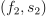.

Returns:
levelSetLevelSet

The intersection between this LevelSet and the LevelSet other, i.e. the LevelSet defined as: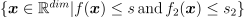.

Examples

>>> import openturns as ot
>>> # First level set
>>> function = ot.SymbolicFunction(['x'], ['3*x-1'])
>>> levelSet1 = ot.LevelSet(function, ot.LessOrEqual(), 0.5)
>>> # Second level set
>>> function = ot.SymbolicFunction(['x'], ['x'])
>>> levelSet2 = ot.LevelSet(function, ot.LessOrEqual(), 0.5)
>>> # Intersection between levelSet1 and levelSet2
>>> intersection = levelSet1.intersect(levelSet2)
>>> # Tests
>>> print([1.0] in intersection)
False
>>> print([0.25] in intersection)
True

join(other)

Return the union with another LevelSet.

Parameters:
otherLevelSet

A LevelSet defined by.

Returns:
levelSetLevelSet

The union between this LevelSet and the LevelSet other, i.e. the LevelSet defined as: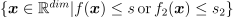.

Examples

>>> import openturns as ot
>>> # First level set
>>> function = ot.SymbolicFunction(['x'], ['3*x-1'])
>>> levelSet1 = ot.LevelSet(function, ot.LessOrEqual(), 0.0)
>>> # Second level set
>>> function = ot.SymbolicFunction(['x'], ['x'])
>>> levelSet2 = ot.LevelSet(function, ot.LessOrEqual(), 0.0)
>>> # Union between levelSet1 and levelSet2
>>> union = levelSet1.join(levelSet2)
>>> # Tests
>>> print([0.5] in union)
False
>>> print([0.25] in union)
True

setFunction(function)

Set the function defining the level set.

Parameters:
functionFunction

A function such that:defining the LevelSet.

Examples

>>> import openturns as ot
>>> levelSet = ot.LevelSet()
>>> function = ot.SymbolicFunction(['x'], ['3*x-1'])
>>> levelSet.setFunction(function)

setLevel(level)

Set the level defining the level set.

Parameters:
levelfloat

Leveldefining the LevelSet.

Examples

>>> import openturns as ot
>>> levelSet = ot.LevelSet()
>>> levelSet.setLevel(3.0)

setLowerBound(bound)

Set the lower bound of the bounding box.

Parameters:
boundsequence of floats

Lower bound of the bounding box of the level set. It allows one to clip the level set.

setName(name)

Accessor to the object’s name.

Parameters:
namestr

The name of the object.

setOperator(op)

Operator accessor.

Parameters:
opComparisonOperator

Comparison operator against the level.

Accessor to the object’s shadowed id.

Parameters:
idint

Internal unique identifier.

setUpperBound(bound)

Set the upper bound of the bounding box.

Parameters:
boundsequence of floats

Upper bound of the bounding box of the level set. It allows one to clip the level set.

setVisibility(visible)

Accessor to the object’s visibility state.

Parameters:
visiblebool

Visibility flag.

## Examples using the class¶Draw minimum volume level sets

Draw minimum volume level sets